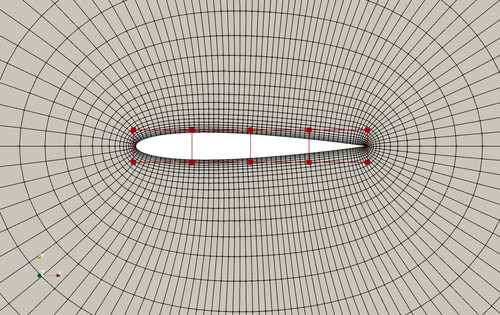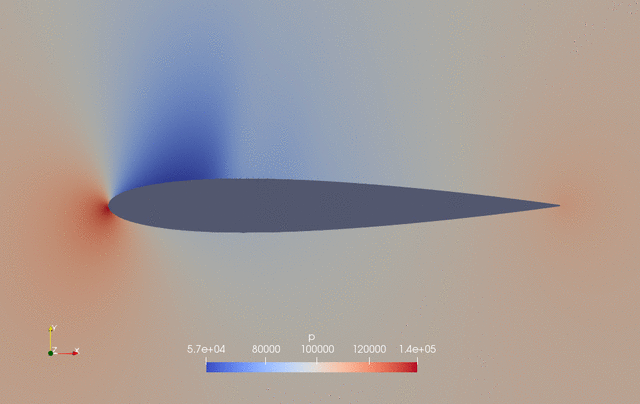The following is an aerodynamic shape optimization case for the NACA0012 airfoil in transonic conditions.

```Case: Airfoil aerodynamic optimization
Geometry: NACA0012
Objective function: Drag coefficient (CD)
Lift coefficient (CL): 0.5
Design variables: 20 free-form deformation (FFD) points moving in the y direction, one angle of attack
Constraints: Symmetry, volume, thickness, and lift constraints (total number: 34)
Mach number: 0.7 (238 m/s)
Reynolds number: 15.9 million
Mesh cells: ~4,500
Solver: DARhoSimpleCFoam
```Fig. 1. Mesh and FFD points for the NACA0012 airfoil

The “runScript.py” is similar to the one used in the NACA0012 subsonic case with the following exceptions:

• We use “DARhoSimpleCFoam”, which is an OpenFOAM built-in compressible flow solver with the “SIMPLEC” algorithm that is suitable for transonic conditions.

• The far field velocity is 238 m/s with Mach number of 0.7.

• We use special treatment for the preconditioner matrix to improve the convergence of adjoint linear equation by setting “transonicPCOption”: 1. This option is only needed for transonic conditions.

To run this case, first download tutorials and untar it. Then go to tutorials-main/NACA0012_Airfoil/transonic and run the “preProcessing.sh” script to generate the mesh:

```./preProcessing.sh
```

Then, use the following command to run the optimization with 4 CPU cores:

```mpirun -np 4 python runScript.py 2>&1 | tee logOpt.txt
```

The case ran for 50 steps and took about 25 minutes using Intel 3.0 GHz CPU with 4 cores. According to “logOpt.txt” and “opt_SLSQP.txt”, the initial drag is 0.029768872 and the optimized drag is 0.023071713 with a drag reduction of 22.5%.

The evolution of pressure and shape during the optimization is as follows.Fig. 2. Pressure and shape evolution during the optimization process